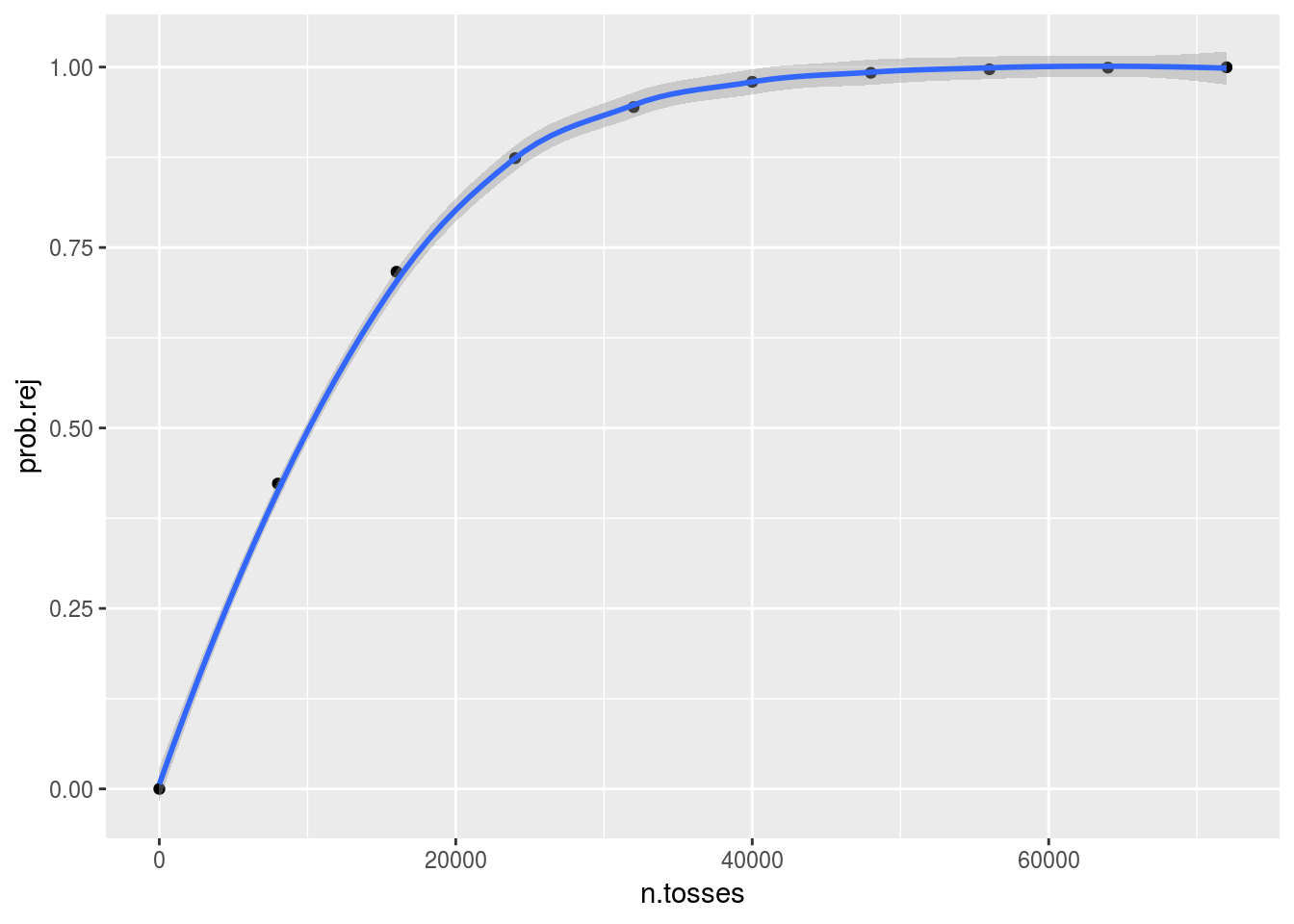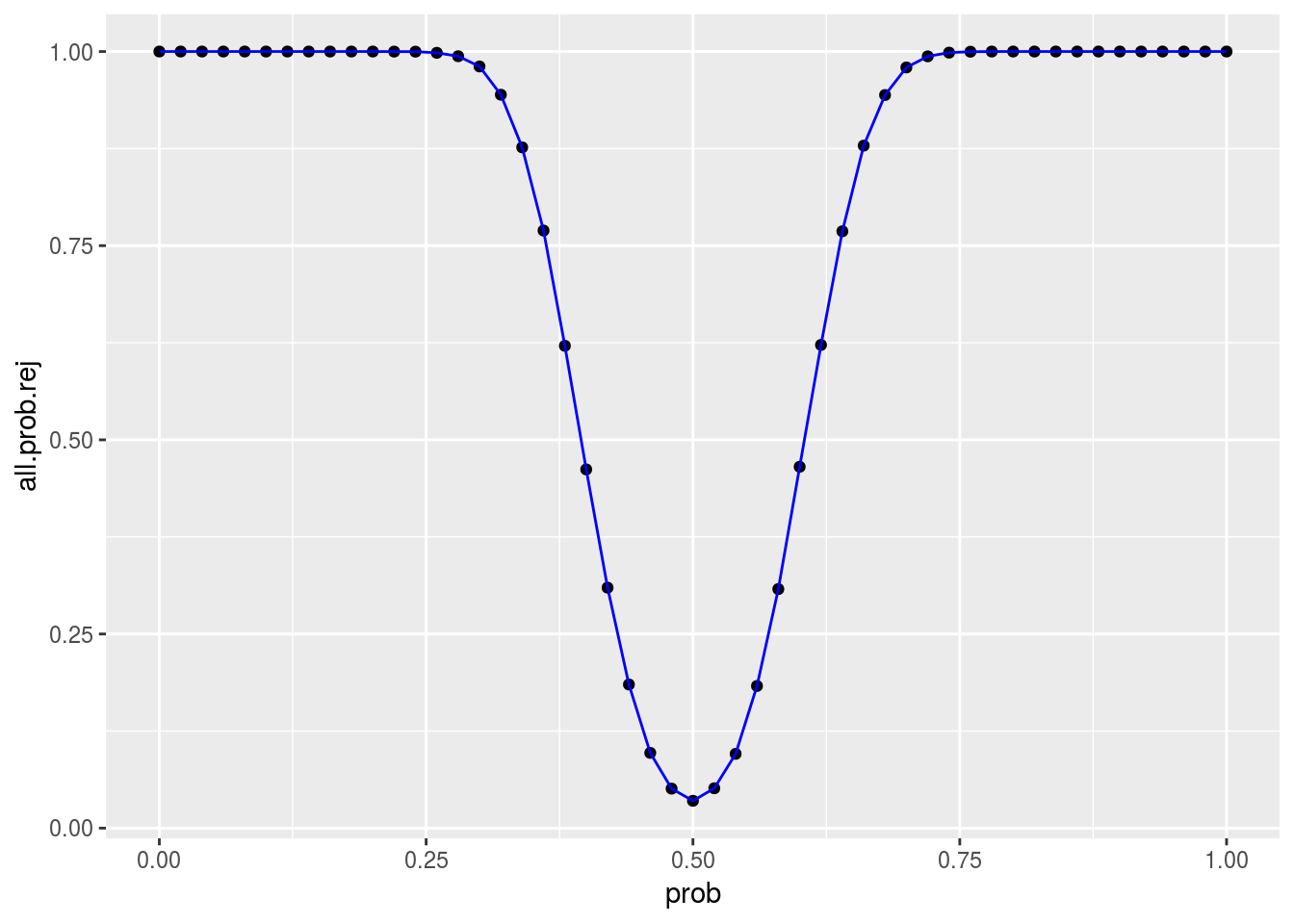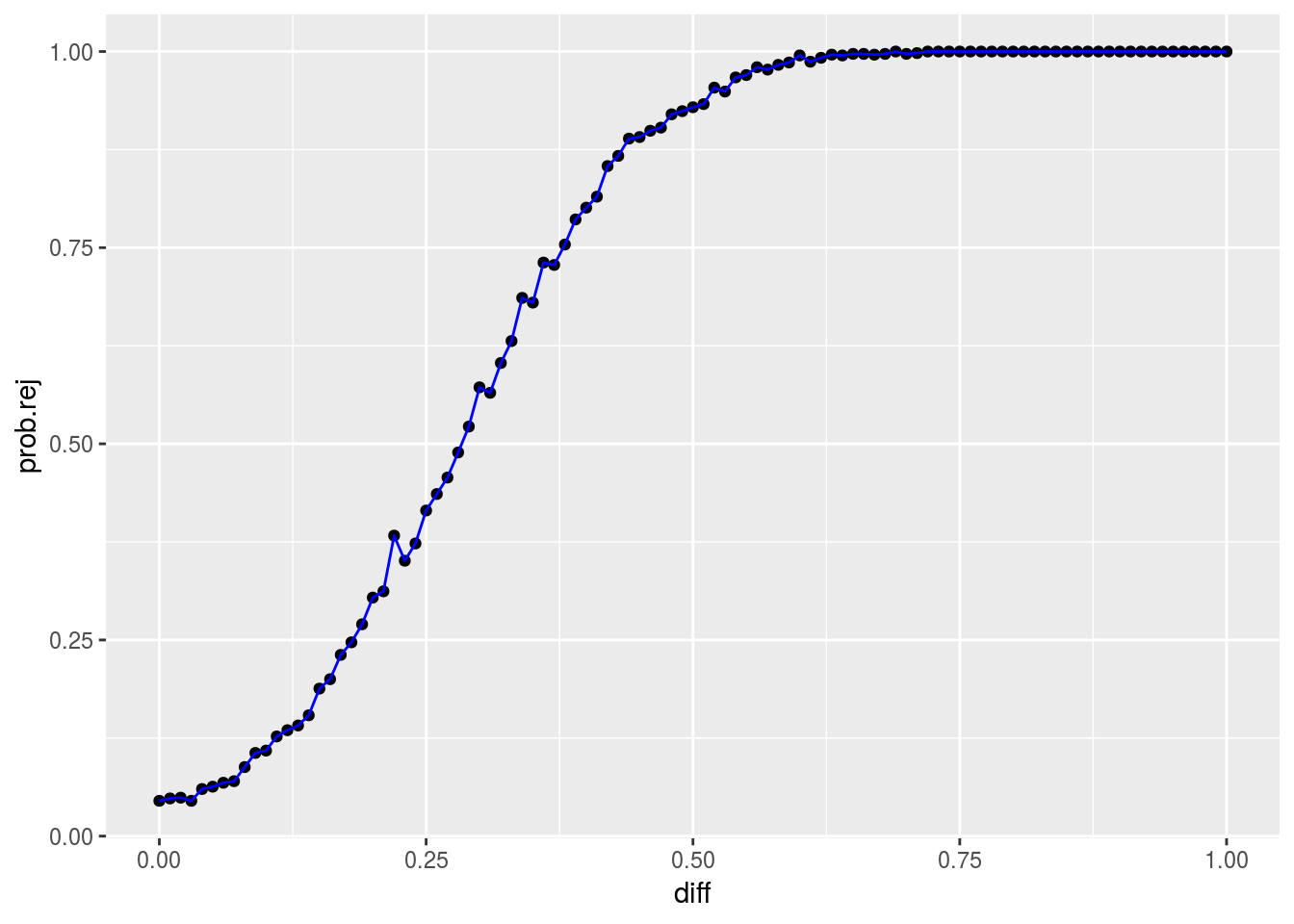### Problem 1

As we saw in lecture, the probability of obtaining a low p-value varies with the size of the sample, even if the null hypothesis is false.

In the problem, we are considering the probability of obtaining a low p-value when tossing a biased coin that comes up Heads 51% of the time.

Plot the probability of obtaining a p-value of 5% or less vs. the size of the sample for this situation. The null hypothesis should be that the coin is fair.

Use simulation to obtain the probability of obtaining a p-value of 5% or less (as in lecture – you can use much of the code in lecture together with sapply).

#### Solution

p.val.r <- function(n.tosses, prob){
s1 <- rbinom(n = 1, size = n.tosses, prob = prob)
p.val <- 2 * pbinom(q = 0.5*n.tosses - abs(0.5*n.tosses - s1), size = n.tosses, prob = 0.5)
return(p.val)
}

mean.p.val.r <- function(n.tosses, prob){
all.p.vals <- replicate(50000, p.val.r(n.tosses, prob))
return(mean(all.p.vals < 0.05))
}

all.n.tosses <- seq(1,80000,8000)
all.probs <- sapply(all.n.tosses, FUN = mean.p.val.r, 0.51)

toss.probs <- data.frame(n.tosses = all.n.tosses, prob.rej = all.probs)
ggplot(toss.probs, mapping = aes(x = n.tosses, y = prob.rej)) + geom_point() + geom_smooth(method = "loess")### Problem 2

The probability of obtaining a low p-value also varies with how different the null hypothesis is from reality.

For 100 trials (i.e., the coin is tossed 100 times and we tally the number of Heads), plot the probability of obtaining a p-value under 5% vs. the probability of the coin’s coming up Heads. The null hypothesis should be that the coin is fair.

#### Solution

mean.p.val.r.2 <- function(prob, n.tosses){
all.p.vals <- replicate(50000, p.val.r(n.tosses, prob))
return(mean(all.p.vals < 0.05))
}

all.probs <- seq(0, 1, 0.02)
all.prob.rej <- sapply(all.probs, FUN = mean.p.val.r.2, 100)

toss.probs <- data.frame(prob = all.probs, prob.rej = all.prob.rej)
ggplot(toss.probs, mapping = aes(x = prob, y = all.prob.rej)) + geom_point() + geom_line(color = "blue")### Problem 3

Now, consider comparing two samples from Gaussian distributions with unknown means and variances.

Plot the probability of obtaining a p-value of 5% or less vs the difference between the means. You can set the standard deviations to 1.0. The null hypothesis should be that there is no difference between the true means.

You can use much of the code from the Finches lecture.

#### Solution

p.val.r.3 <- function(diff){
s1 <- rnorm(n = 100, mean = 0, sd = 1)
s2 <- rnorm(n = 100, mean = diff, sd = 1)
return(t.test(s1, s2)\$p.value)
}

mean.p.val.r.3 <- function(diff){
all.p.vals <- replicate(1000, p.val.r.3(diff))
return(mean(all.p.vals < 0.05))
}

all.diffs <- seq(0, 1, 0.01)
all.prob.rej <- sapply(all.diffs, FUN = mean.p.val.r.3)

diff.probs <- data.frame(diff = all.diffs, prob.rej = all.prob.rej)
ggplot(diff.probs, mapping = aes(x = diff, y = prob.rej)) + geom_point() + geom_line(color = "blue")